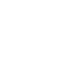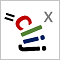# CLIJ2GPU accelerated image processing for everyone

CLIJ2 home

## gammaCorrectionApplies a gamma correction to an image.

Therefore, all pixels x of the Image X are normalized and the power to gamma g is computed, before normlization is reversed (^ is the power operator):f(x) = (x / max(X)) ^ gamma * max(X)

Category: Filter

### Usage in ImageJ macro

``````Ext.CLIJx_gammaCorrection(Image input, Image destination, Number gamma);
``````

Imprint